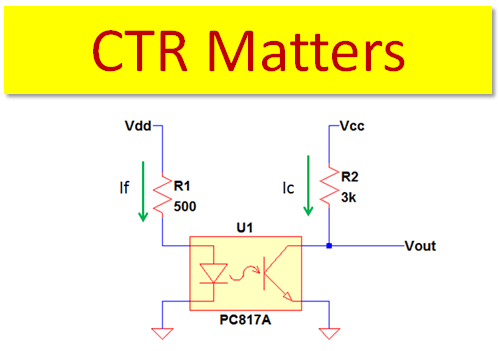# Disadvantage of using Optocoupler with Low CTR for Switch Operation

In optocoupler, CTR stands for current transfer ratio. This is comparable to the current gain beta in BJT. This is the most important parameters of an optocoupler to function normally. There are several factors that affect the CTR, read here. In this article we are going to focus what is the disadvantage of using Optocoupler with low CTR particularly in switch operation. Mathematically, CTR isWhere Ic and If are collector and forward currents respectively. Normally at linear operation, above equation is true. However when the optocoupler circuit saturates, it is not anymore valid to use to get the exact collector current. At saturation the collector current is only limited with the collector resistor. In order for the optocoupler circuit to saturate the circuit CTR must be less than the device CTR. Circuit CTR is the ratio of the collector to forward current of the circuit. On the other hand the device CTR is the current transfer ratio defined in the datasheet. In linear operation these two are equal. The circuit CTR will always follow the device CTR as above equation.

Now, what is the disadvantage of using optocoupler with low CTR in switch operation? As metioned in the above paragraph, at saturation the circuit CTR is less than the device CTR. Expresing it mathematically,Circuit CTR is the ratio of the collector to the forward current of the optocoupler circuit. So we can change the above equation as belowBased on the above equation, when the collector current increases the circuit CTR will increase. In switch design, the collector current is mostly higher since the optocoupler is operating at saturation. If your device CTR is not high enough there is a tendency that the operation will go out from saturation. What will happen if the computed circuit CTR is equal to the device CTR? The opto will not anymore saturate. In actual design, the circuit CTR is purposely set very small compared to the device CTR (linear CTR). By doing this you are erasing the gray areas in between.

Another big thing is that the device CTR will degrade with higher temperature and aging. Say the CTR of the device at nominal ambient temperature is 100%, this may become 60% considering the effect of temperature and aging (lifetime de-rating). This is the main disadvantage of using optocoupler with low CTR specifically in switch operation. For complete discussion how CTR affected by temperature and aging read this.

## One comment

1. Midhul says:

In which basis ctr is categoried??
What are their applications?

This site uses Akismet to reduce spam. Learn how your comment data is processed.